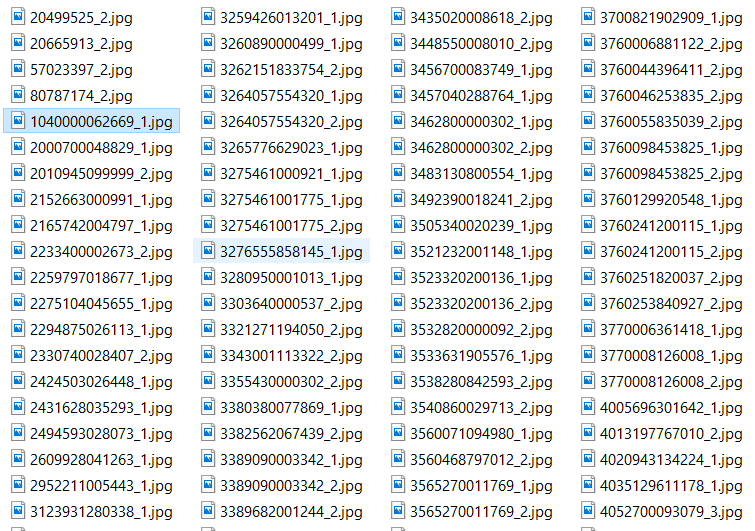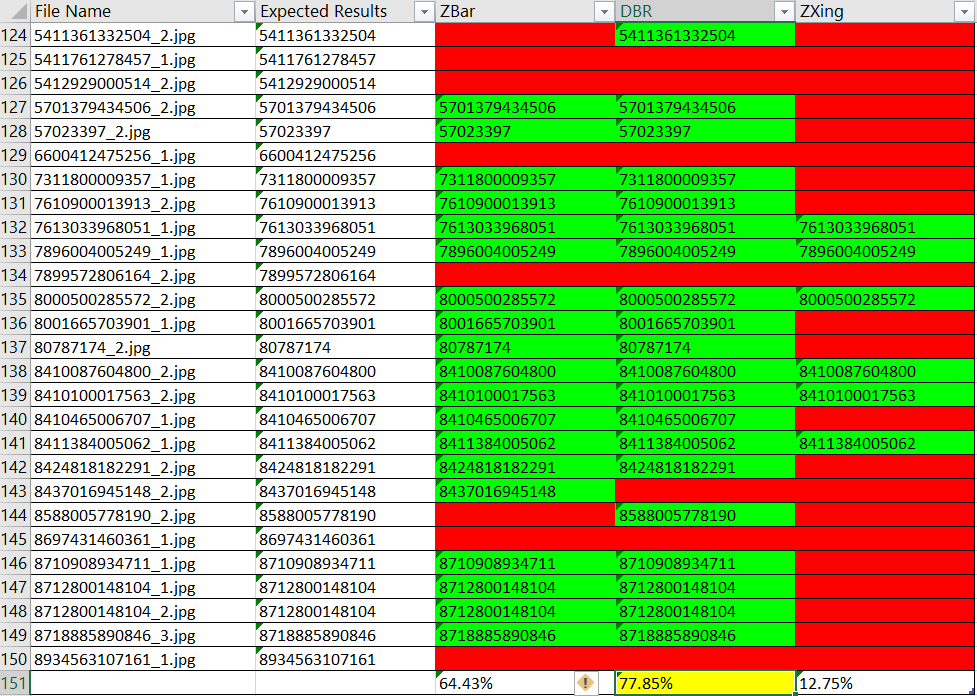×

# How to Benchmark Barcode SDK Performance in Python

On StackOverflow, you may have seen this kind of question: why some open-source barcode SDK failed to decode some barcode? Usually, there are three optional workarounds: improve the input image quality, improve the barcode algorithm, or find a better barcode SDK.  ZXing and ZBar are probably the most-welcomed open-source barcode SDKs, but their algorithms rarely enhanced over the past years. Why not use commercial barcode SDK if free SDK is not ideal? Last week, I published an article demonstrating how to use ZXing and ZBar in Python. In this article, I will show you how to use Dynamsoft Barcode Reader in Python, as well as compare its performance to ZXing and ZBar.

## Using Dynamsoft Barcode Reader in Python

Install the Python package:

``````pip install dbr==7.2.2.3
``````

Here is the quick usage:

``````from dbr import DynamsoftBarcodeReader

try:

start = time.time()
elapsed_time = time.time() - start
textResults = dbr_results["TextResults"]
resultsLength = len(textResults)
if resultsLength > 0:
for textResult in textResults:
print(textResult["BarcodeFormatString"])
print('Dynamsoft Barcode Reader: {}. Elapsed time: {}ms'.format(textResult["BarcodeText"], int(elapsed_time * 1000)))

return textResults
else:
print("DBR failed to decode {}".format(filename))
except Exception as err:
print("DBR failed to decode {}".format(filename))

``````

To use the commercial barcode SDK, you have to get a free trial license.

## How to Get the Recognition Rate Based on the DatasetCheck out the image dataset, and you will find the barcode results are in the file names, with which we can verify whether the decoded results are correct or not.

Get the file list from a directory:

``````import os
files = os.listdir(directory)
``````

Filter the image files by checking the suffix:

``````files = [f for f in files if f.endswith('.jpg') or f.endswith('.png')]
``````

Split the file name to get the expected result:

``````expected_result = filename.split('_')
``````

Increase the count when barcode results match the expected results:

``````if r1 == expected_result:
zbar_count += 1

if r2 == expected_result:
dbr_count += 1

if r3 == expected_result:
zxing_count += 1
``````

Calculate the recognition rate of ZBar, Dynamsoft Barcode Reader, and ZXing:

``````total_count = len(files)

zbar_rate = zbar_count * 100 / total_count
print('ZBar recognition rate: {0:.2f}%'.format(zbar_rate))

dbr_rate = dbr_count * 100 / total_count
print('DBR recognition rate: {0:.2f}%'.format(dbr_rate))

zxing_rate = zxing_count * 100 / total_count
print('ZXing recognition rate: {0:.2f}%'.format(zxing_rate))
``````

## How to Read and Write Excel File in Python

After benchmarking the barcode SDK performance, the final step is to save the results to an Excel file. You can select a Python package from http://www.python-excel.org/.

I picked openpyxl:

``````pip install openpyxl
``````

Create a new workbook or load a workbook from an existing Excel file:

``````from openpyxl import utils
from openpyxl import Workbook
from openpyxl.styles import Color, PatternFill
import os

def get_workbook(wb_name):

if os.path.isfile(wb_name):
else:
wb = Workbook()
ws = wb.active
ws.title = 'Recognition Rate'
ws['A1'] = 'File Name'
# Set column width
ws.column_dimensions[utils.get_column_letter(1)].width = 25
ws['B1'] = 'Expected Results'
ws.column_dimensions[utils.get_column_letter(2)].width = 20
ws['C1'] = 'ZBar'
ws.column_dimensions[utils.get_column_letter(3)].width = 20
ws['D1'] = 'DBR'
ws.column_dimensions[utils.get_column_letter(4)].width = 20
ws['E1'] = 'ZXing'
ws.column_dimensions[utils.get_column_letter(5)].width = 20
return wb
``````

Set the value and change the fill color for worksheet cell:

``````red = PatternFill(start_color='FFFF0000',
end_color='FFFF0000',
fill_type='solid')

green = PatternFill(start_color='FF00FF00',
end_color='FF00FF00',
fill_type='solid')

def update_row(wb, row_index, filename=None, expected_results=None, zbar_results=None, dbr_results=None, ZXing_results=None):
ws = wb.active
row = ws[row_index]
row.value = filename
row.value = expected_results
if zbar_results != None:
row.value = zbar_results
if zbar_results == expected_results:
row.fill = green
else:
row.fill = red

if dbr_results != None:
row.value = dbr_results
if dbr_results == expected_results:
row.fill = green
else:
row.fill = red

if ZXing_results != None:
row.value = ZXing_results
if ZXing_results == expected_results:
row.fill = green
else:
row.fill = red
``````

Save the workbook to a .xlsx file:

``````def save_workbook(wb, wb_name):
if wb != None:
wb.save(wb_name)
``````

Now run the Python app to generate the benchmark file:

``````python app.py -d D:\\python-zxing-zbar-dbr\\dataset
``````## Source Code

https://github.com/yushulx/python-zxing-zbar-dbr